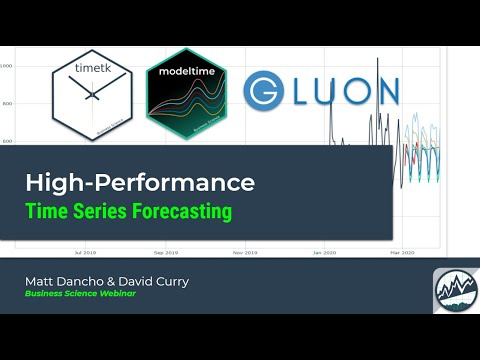# modeltime.gluonts

Deep Learning for Time Series, simplified.

Important: This package is exprimental. Functions may change until the package matures.

Modeltime GluonTS integrates the Python GluonTS Deep Learning Library, making it easy to develop forecasts using Deep Learning for those that are comfortable with the Modeltime Forecasting Workflow.## GluonTS in R

Using `deep_ar()`, which connects to `GluonTS DeepAREstimator()`.

``````library(modeltime.gluonts)
library(tidymodels)
library(tidyverse)

# Fit a GluonTS DeepAR Model
model_fit_deepar <- deep_ar(
id                    = "id",
freq                  = "M",
prediction_length     = 24,
lookback_length       = 36,
epochs                = 10,
num_batches_per_epoch = 50,
learn_rate            = 0.001,
num_layers            = 2,
dropout               = 0.10
) %>%
set_engine("gluonts_deepar") %>%
fit(value ~ ., training(m750_splits))

# Forecast with 95% Confidence Interval
modeltime_table(
model_fit_deepar
) %>%
modeltime_calibrate(new_data = testing(m750_splits)) %>%
modeltime_forecast(
new_data      = testing(m750_splits),
actual_data   = m750,
conf_interval = 0.95
) %>%
plot_modeltime_forecast(.interactive = FALSE)``````## Installation

`modeltime.gluonts` is currently available on GitHub only. Not on CRAN yet.

``remotes::install_github("business-science/modeltime.gluonts")``

## Required: Python Environment Setup

Important: Use `install_gluonts()` to set up the “r-gluonts” `python` environment used by `modeltime.gluonts`. You only need to do this once, when you first set up the package.

``````# GluonTS Installation
# - This sets up the Python Environment
# - Only need to run 1-time, then you're set!
install_gluonts()``````

# Learning MoreMy Talk on High-Performance Time Series Forecasting

Time series is changing. Businesses now need 10,000+ time series forecasts every day. This is what I call a High-Performance Time Series Forecasting System (HPTSF) - Accurate, Robust, and Scalable Forecasting.

High-Performance Forecasting Systems will save companies MILLIONS of dollars. Imagine what will happen to your career if you can provide your organization a “High-Performance Time Series Forecasting System” (HPTSF System).

I teach how to build a HPTFS System in my High-Performance Time Series Forecasting Course. If interested in learning Scalable High-Performance Forecasting Strategies then take my course. You will learn:

• Time Series Machine Learning (cutting-edge) with `Modeltime` - 30+ Models (Prophet, ARIMA, XGBoost, Random Forest, & many more)
• NEW - Deep Learning with `GluonTS` (Competition Winners)
• Time Series Preprocessing, Noise Reduction, & Anomaly Detection
• Feature engineering using lagged variables & external regressors
• Hyperparameter Tuning
• Time series cross-validation
• Ensembling Multiple Machine Learning & Univariate Modeling Techniques (Competition Winner)
• Scalable Forecasting - Forecast 1000+ time series in parallel
• and more.

Unlock the High-Performance Time Series Forecasting Course